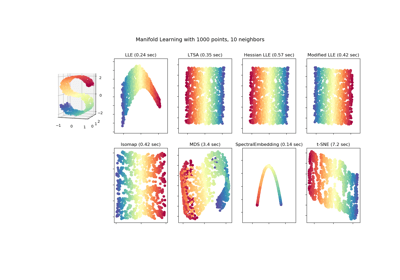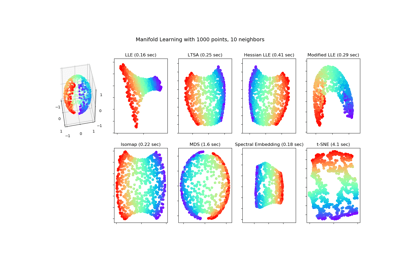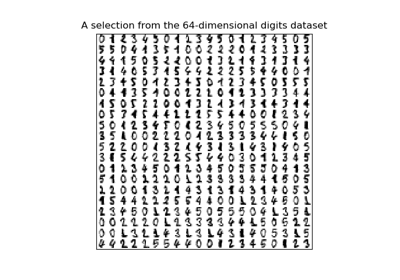/scikit-learn

sklearn.manifold.Isomap

class sklearn.manifold.Isomap(n_neighbors=5, n_components=2, eigen_solver=’auto’, tol=0, max_iter=None, path_method=’auto’, neighbors_algorithm=’auto’, n_jobs=None) [source]

Isomap Embedding

Non-linear dimensionality reduction through Isometric Mapping

Read more in the User Guide.

Parameters: n_neighbors : integer number of neighbors to consider for each point. n_components : integer number of coordinates for the manifold eigen_solver : [‘auto’|’arpack’|’dense’] ‘auto’ : Attempt to choose the most efficient solver for the given problem. ‘arpack’ : Use Arnoldi decomposition to find the eigenvalues and eigenvectors. ‘dense’ : Use a direct solver (i.e. LAPACK) for the eigenvalue decomposition. tol : float Convergence tolerance passed to arpack or lobpcg. not used if eigen_solver == ‘dense’. max_iter : integer Maximum number of iterations for the arpack solver. not used if eigen_solver == ‘dense’. path_method : string [‘auto’|’FW’|’D’] Method to use in finding shortest path. ‘auto’ : attempt to choose the best algorithm automatically. ‘FW’ : Floyd-Warshall algorithm. ‘D’ : Dijkstra’s algorithm. neighbors_algorithm : string [‘auto’|’brute’|’kd_tree’|’ball_tree’] Algorithm to use for nearest neighbors search, passed to neighbors.NearestNeighbors instance. n_jobs : int or None, optional (default=None) The number of parallel jobs to run. None means 1 unless in a joblib.parallel_backend context. -1 means using all processors. See Glossary for more details. embedding_ : array-like, shape (n_samples, n_components) Stores the embedding vectors. kernel_pca_ : object KernelPCA object used to implement the embedding. training_data_ : array-like, shape (n_samples, n_features) Stores the training data. nbrs_ : sklearn.neighbors.NearestNeighbors instance Stores nearest neighbors instance, including BallTree or KDtree if applicable. dist_matrix_ : array-like, shape (n_samples, n_samples) Stores the geodesic distance matrix of training data.

References

  Tenenbaum, J.B.; De Silva, V.; & Langford, J.C. A global geometric framework for nonlinear dimensionality reduction. Science 290 (5500)

Examples

>>> from sklearn.datasets import load_digits
>>> from sklearn.manifold import Isomap
>>> X, _ = load_digits(return_X_y=True)
>>> X.shape
(1797, 64)
>>> embedding = Isomap(n_components=2)
>>> X_transformed = embedding.fit_transform(X[:100])
>>> X_transformed.shape
(100, 2)

Methods

 fit(X[, y]) Compute the embedding vectors for data X fit_transform(X[, y]) Fit the model from data in X and transform X. get_params([deep]) Get parameters for this estimator. reconstruction_error() Compute the reconstruction error for the embedding. set_params(**params) Set the parameters of this estimator. transform(X) Transform X.
__init__(n_neighbors=5, n_components=2, eigen_solver=’auto’, tol=0, max_iter=None, path_method=’auto’, neighbors_algorithm=’auto’, n_jobs=None) [source]
fit(X, y=None) [source]

Compute the embedding vectors for data X

Parameters: X : {array-like, sparse matrix, BallTree, KDTree, NearestNeighbors} Sample data, shape = (n_samples, n_features), in the form of a numpy array, precomputed tree, or NearestNeighbors object. y : Ignored self : returns an instance of self.
fit_transform(X, y=None) [source]

Fit the model from data in X and transform X.

Parameters: X : {array-like, sparse matrix, BallTree, KDTree} Training vector, where n_samples in the number of samples and n_features is the number of features. y : Ignored X_new : array-like, shape (n_samples, n_components)
get_params(deep=True) [source]

Get parameters for this estimator.

Parameters: deep : boolean, optional If True, will return the parameters for this estimator and contained subobjects that are estimators. params : mapping of string to any Parameter names mapped to their values.
reconstruction_error() [source]

Compute the reconstruction error for the embedding.

Returns: reconstruction_error : float

Notes

The cost function of an isomap embedding is

E = frobenius_norm[K(D) - K(D_fit)] / n_samples

Where D is the matrix of distances for the input data X, D_fit is the matrix of distances for the output embedding X_fit, and K is the isomap kernel:

K(D) = -0.5 * (I - 1/n_samples) * D^2 * (I - 1/n_samples)

set_params(**params) [source]

Set the parameters of this estimator.

The method works on simple estimators as well as on nested objects (such as pipelines). The latter have parameters of the form <component>__<parameter> so that it’s possible to update each component of a nested object.

Returns: self
transform(X) [source]

Transform X.

This is implemented by linking the points X into the graph of geodesic distances of the training data. First the n_neighbors nearest neighbors of X are found in the training data, and from these the shortest geodesic distances from each point in X to each point in the training data are computed in order to construct the kernel. The embedding of X is the projection of this kernel onto the embedding vectors of the training set.

Parameters: X : array-like, shape (n_samples, n_features) X_new : array-like, shape (n_samples, n_components)

Examples using sklearn.manifold.IsomapComparison of Manifold Learning methodsManifold Learning methods on a severed sphereManifold learning on handwritten digits: Locally Linear Embedding, Isomap…

© 2007–2018 The scikit-learn developers